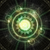0

# sort performance

ccc 3 years ago updated by Pavel Holoborodko 3 years ago

what is the performance of sort function of this toolbox?

Are there any comparison studies?+1

We use quicksort algorithm, which is pretty much de-facto standard in all programming languages.

If we compare sort speed of our toolbox and VPA:

`>> A = vpa(rand(10000,1));>> tic; sort(A); toc;Elapsed time is 0.065205 seconds.>> A = rand(10000,1,'mp');>> tic; sort(A); toc;Elapsed time is 0.003493 seconds.`

So, our toolbox is ~19 times faster.+1
`>> A = vpa(rand(10000,1));>> tic; sort(A); toc;Elapsed time is 0.065205 seconds.>> A = rand(10000,1,'mp');>> tic; sort(A); toc;Elapsed time is 0.003493 seconds.`